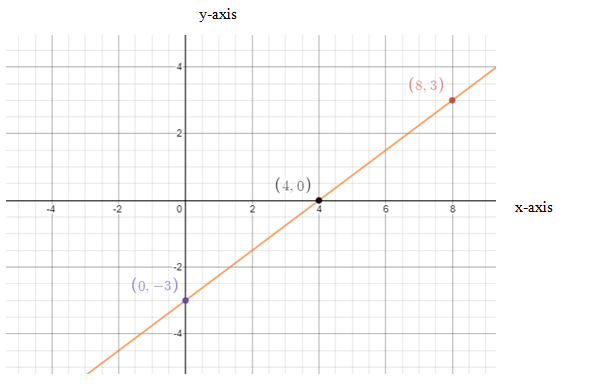# To find the slope and y-intercept of the line and then draw its graph### Single Variable Calculus: Concepts...

4th Edition
James Stewart
Publisher: Cengage Learning
ISBN: 9781337687805### Single Variable Calculus: Concepts...

4th Edition
James Stewart
Publisher: Cengage Learning
ISBN: 9781337687805

#### Solutions

Chapter B, Problem 27E
To determine

## To calculate: To find the slope and y-intercept of the line and then draw its graph

Expert Solution

Slope of line is 34 and y-intercept of line is 3

### Explanation of Solution

Given information: Equation is 3x4y=12

Formula Used:

Slope intercept form of line is

y=mx+c

Where,

m= Slope

c= y-intercept

Calculation:

Equation of line is given as follows:

3x4y=12

Rewriting the equation as follows:

4y=3x12y=34x124y=34x3

Comparing the above equation with slope-intercept form of line y=mx+c ,

m=34c=3

Finding the points that lie on equation:

When x=0

y=34(0)3y=3

When x=4

y=34(4)3y=33y=0

When x=8

y=34(8)3y=63y=3

Thus, (0,3),(4,0),(8,3) are the solution of equation and must lie on the equation.

Plotting the points on graph,Conclusion:

Hence, slope of line 34 and y-intercept of line is 3

### Have a homework question?

Subscribe to bartleby learn! Ask subject matter experts 30 homework questions each month. Plus, you’ll have access to millions of step-by-step textbook answers!